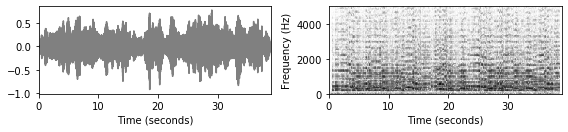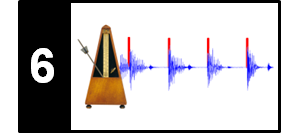# Onset Detection

Following Section 6.1 of [Müller, FMP, Springer 2015], we introduce in this notebook the task referred to as onset detection. An overview of onset detection methods can also be found in the following articles:

• Juan Pablo Bello, Laurent Daudet, Samer A. Abdallah, Chris Duxbury, Mike E. Davies, and Mark B. Sandler: A Tutorial on Onset Detection in Music Signals. IEEE Transaction on Speech and Audio Processing 13(5-2), 2005, pp. 1035–1047.
• Simon Dixon: Onset Detection Revisited. Proceedings of the International Conference on Digital Audio Effects (DAFx), 2006, pp. 133–137.

## Musical Onsets¶

The notion of a musical onset can be rather vague and is related to other concepts such as attacks or transients. When playing a note on an instrument such as a piano, there is often a sudden increase of energy at the beginning of a musical tone. The attack of a note refers to the phase where the sound builds up, which typically goes along with a sharply increasing amplitude envelope. The related concept of a transient refers to a noise-like sound component of short duration and high amplitude typically occurring at the beginning of a musical tone or a more general sound event. As opposed to the attack and transient, the onset of a note refers to the single instant (rather than a period) that marks the beginning of the transient, or the earliest time point at which the transient can be reliably detected. This is illustrated by the following figure.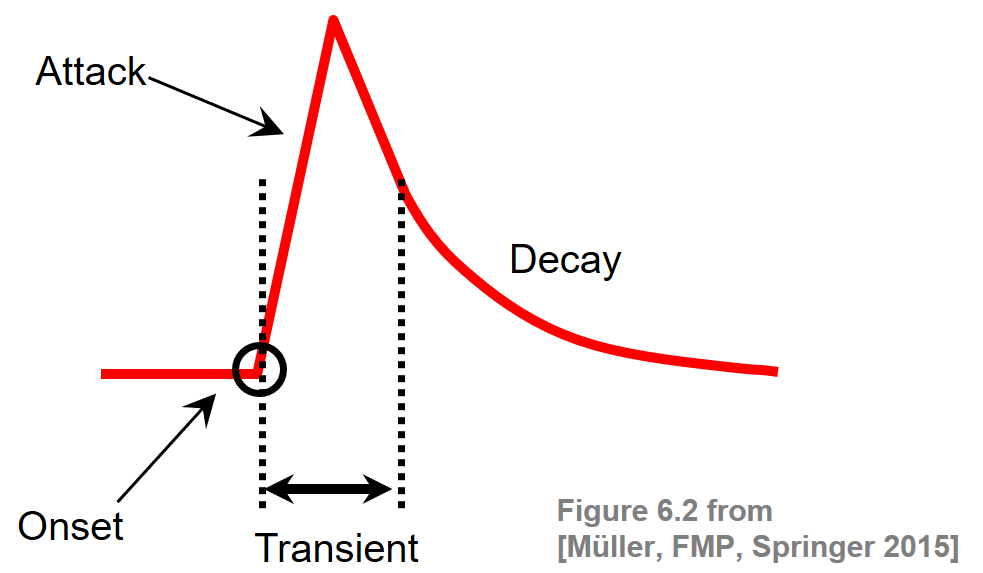Intuitively speaking, onset detection is the task of determining the starting times of notes or other musical events as they occur in a music recording. To detect note onsets in the signal, the general idea is to capture sudden changes that often mark the beginning of transient regions. For notes that have a pronounced attack phase, onset candidates may be determined by locating time positions where the signal's amplitude envelope starts increasing. In the following figure, we show the waveform and the spectrogram of a click sound as well as of a piano sound (playing the note C4):

In :
import os, sys
import sys
import numpy as np
from scipy import signal
from  matplotlib import pyplot as plt
import librosa
import IPython.display as ipd
import pandas as pd
sys.path.append('..')
import libfmp.b
import libfmp.c2
import libfmp.c6

%matplotlib inline

def plot_wav_spectrogram(fn_wav, xlim=None, audio=True):
"""Plot waveform and computed spectrogram and may display audio
Notebook: C6/C6S1_OnsetDetection.ipynb
"""
Fs = 22050
x, Fs = librosa.load(fn_wav, Fs)
plt.figure(figsize=(8,2))
ax = plt.subplot(1,2,1)
libfmp.b.plot_signal(x, Fs, ax=ax)
if xlim!=None: plt.xlim(xlim)
ax = plt.subplot(1,2,2)
N, H = 512, 256
X = librosa.stft(x, n_fft=N, hop_length=H, win_length=N, window='hanning')
Y = np.log(1 + 10 * np.abs(X))
libfmp.b.plot_matrix(Y, Fs=Fs/H, Fs_F=N/Fs, ax=[ax], colorbar=False)
plt.ylim([0,5000])
if xlim is not None: plt.xlim(xlim)
plt.tight_layout()
plt.show()
if audio: ipd.display(ipd.Audio(x, rate=Fs))

fn_wav = os.path.join('..', 'data', 'C6', 'FMP_C6_F04_Impulse.wav')
plot_wav_spectrogram(fn_wav)

fn_wav = os.path.join('..', 'data', 'C6', 'FMP_C6_F04_NoteC4_Piano.wav')
plot_wav_spectrogram(fn_wav)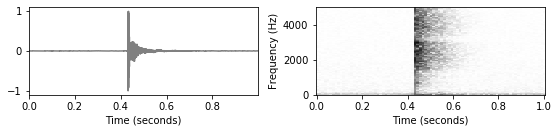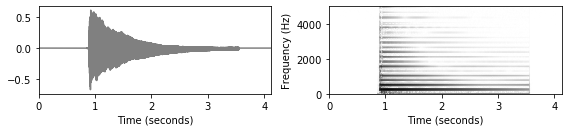When there is no clear attack phase, such as for nonpercussive music with soft onsets and blurred note transitions, the detection of onsets is much more challenging. For example, the waveform of a violin sound may exhibit a slow energy increase rather than an abrupt change as in a piano sound. For soft sounds, it is hard to determine or even to define the exact onset position. This is illustrated by the violin example (sound of C4) of the next figure:

In :
fn_wav = os.path.join('..', 'data', 'C6', 'FMP_C6_F04_NoteC4_Violin.wav')
plot_wav_spectrogram(fn_wav)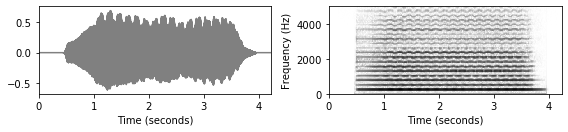The detection of individual note onsets becomes even harder when dealing with complex polyphonic music. Simultaneously occurring sound events may result in masking effects, where no significant changes in the signal's energy are measurable. This is illustrated by the first measures of the third movement of Borodin's String Quartet No. 2.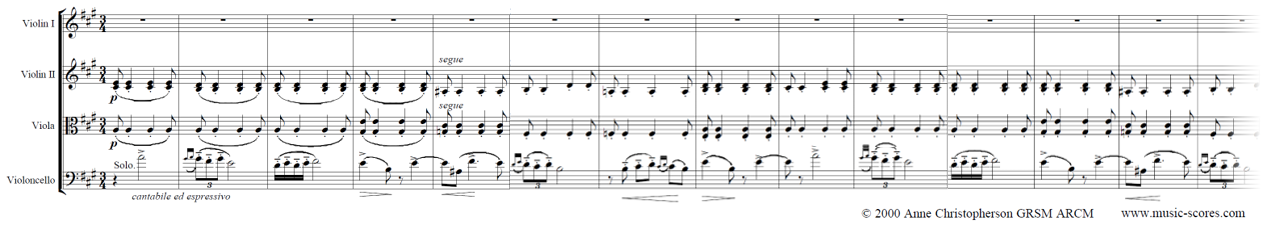In :
#fn_wav = os.path.join('..', 'data', 'C6', 'FMP_C6_Audio_Faure_Op015-01-sec0-12_SMD126.wav')
fn_wav = os.path.join('..', 'data', 'C6', 'FMP_C6_Audio_Borodin-sec39_RWC.wav')
plot_wav_spectrogram(fn_wav)
print('Plot of the first six seconds:')
plot_wav_spectrogram(fn_wav, xlim=[0,6], audio=False)